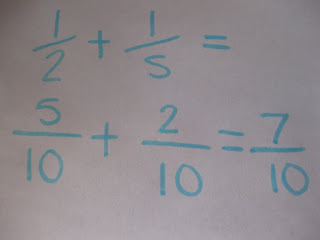### Jordan's Last Fraction Post

Monday, May 11, 2009To add fractions, you must first find the common denominator. This is usually the sum of the two denomainators multiplied. The common denominator below is 10. Then you figure out how many times the denominator of the first fraction goes into the numerator of the fraction below. Then you times that number by the numerator of the top fraction. As shown on the picture, 2 goes into 10 5 times, and 5 times 1 is 5. Use this same rule for the other fraction(s). When you get the numerators of the remaining two fractions, add them to get the final answer.When adding Mixed Numbers, you can add the fractions first. Use the same rule above to do this, except make the sum of the two added fractions into a mixed number. When this is finished just add the whole numbers from the question to the mixed number to get your final answer.

Subtracting Fractions
To subtract fractions, you must again find the common denominator. Then use the same rule that you used for adding fractions. (How many times does the denominator go into the denominator, then times by the numerator) and subtract the wholes.

Multiplying fractions is probably the easiest. All you must do is multiply the numerators and the denominators, then simplify if possible.

Multiplying Mixed Fractions
Same goes for multiplying normal fractions, except you must convert the mixed numbers to improper fractions, then multiply and simplify.

Dividing Fractions
When dividing fractions, you first have to find the reciprocal. This is the fraction on the right flipped upside-down in order to turn a fraction into 1. You then times this reciprocal by the left fraction.

Dividing Mixed Fractions

Same as above.

Problem 2.
It takes 2 1/4 scoops of flour to make one cake.

How many cakes do 15 scoops of flour make?

15 scoops of flour make 6 2/3 cakes.

Problem 3.
One day in Winnipeg with 10 1/2 hours of daylight, it was sunny for 1/3 of that time. For how many hours was it sunny that day?

It was sunny for 3 1/2 hours that day.